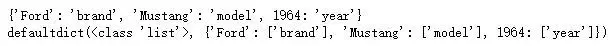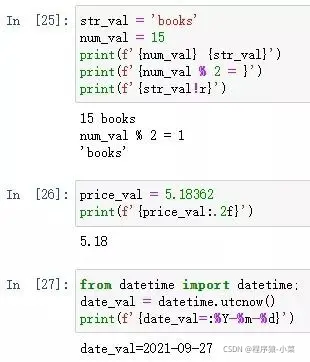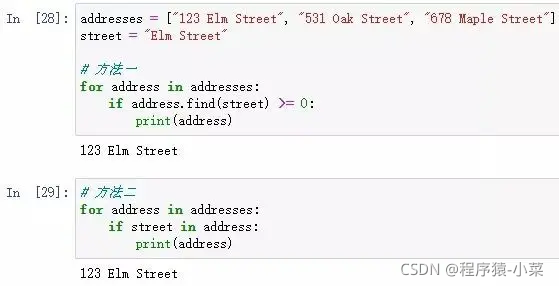# Python小技巧

1. 合并两个或多个字典

from collections import defaultdict

def merge_dicts(*dicts):

mdict = defaultdict(list)

for dict in dicts:

for key in dict:

res[key].append(d[key])

return dict(mdict)

2. 反转字典

my_dict = {

brand: Ford,

model: Mustang,

year: 1964

}

# 方法一

my_inverted_dict_1 = dict(map(reversed, my_dict.items()))

# 方法二

from collections import defaultdict

my_inverted_dict_2 = defaultdict(list)

{my_inverted_dict_2[v].append(k) for k, v in my_dict.items()}

print(my_inverted_dict_1)

print(my_inverted_dict_2)3. 使用 f 字符串格式化字符串

str_val = 'books'

num_val = 15

print(f'{num_val} {str_val}')

print(f'{num_val % 2 = }')

print(f'{str_val!r}')

price_val = 5.18362

print(f'{price_val:.2f}')

from datetime import datetime;

date_val = datetime.utcnow()

print(f'{date_val=:%Y-%m-%d}')4. 检查子串

addresses = [123 Elm Street, 531 Oak Street, 678 Maple Street]

street = Elm Street

# 方法一

# 方法二5. 以字节为单位获取字符串的大小

str1 = hello

str2 = 

def str_size(s):

return len(s.encode('utf-8'))

print(str_size(str1))

print(str_size(str2))6. 检查文件是否存在

# 方法一

import os

exists = os.path.isfile('/path/to/file')

# 方法二

from pathlib import Path

config = Path('/path/to/file')

if config.is_file():

pass

7.解析电子表格

import csv

csv_mapping_list = []

with open(/path/to/data.csv) as my_data:

line_count = 0

if line_count == 0:

else:

row_dict = {key: value for key, value in zip(header, line)}

csv_mapping_list.append(row_dict)

line_count += 1

————————————————